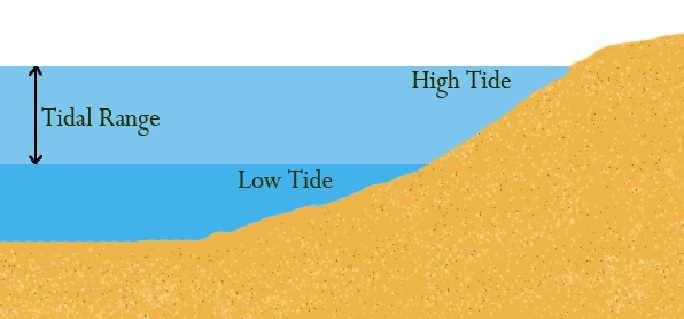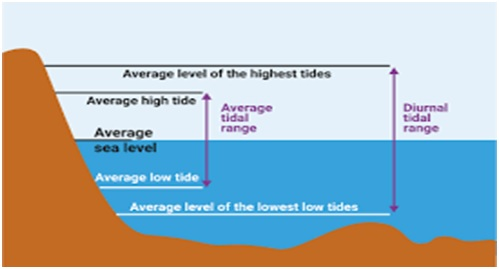What is meaning of Tidal Range? |

# What is meaning of Tidal Range?Written by
###### Tidal Range

Whether a tide is neap or spring (or an intermediate) is determined not by the height of high water but by the difference between the height of high water and the next (or preceding) low water. This is called the tidal range.

##### Note :
• Spring tides have a large range and neap tides a small one.
• The mean sea level remains roughly constant.
• At springs, the high water is very high and the low water very low, while at neaps, the high water is much lower and the low water much higher.
• With the introduction of international standards for electronic charting, there is a trend for all hydrographic authorities to adopt the same terminology for tide levels. Although there are many different tidal height, such as MLW (mean low water), MLLW (mean lower low water), MHW (mean high water) and MHHW (mean higher high water), which are still used on paper charts, the following are understood and becoming more common:

MHWS mean high water springs
MHWN mean high water neaps
MLWS mean low water springs
MLWN – mean low water neaps.

Mean low water (MLW) is the average height of all low waters at a given place. About half of the low waters fall below it, and half above.

Mean low water springs (MLWS), usually shortened to low water springs, is the average level of the low waters that occur at the times of spring tides.

Mean lower low water (MLLW) is the average height of the lower low waters of each tidal day.

Tropic lower low water (TcLLW) is the average height of the lower low waters (or of the single daily low waters if the tide becomes diurnal) that occur when the Moon is near maximum declination and the diurnal effect ismost pronounced. This datum is not in common use as a tidal reference.

Mean lower low water springs (MLLWS) is the average level of the lower of the two low waters on the days of spring tides.

Mean high water springs (MHWS), which is the average level of the high waters that occur at the time of spring tides;

Mean higher high water (MHHW), which is the average height of the higher high waters of each tidal day.

Tropic higher high water (TcHHW), which is the average height of the higher high waters (or the single daily high waters if the tide becomes diurnal) that occur when the Moon is near maximum declination and the diurnal effect is most pronounced.

Approximately, spring tides will be 25% greater than the mean range and neap tides will be 25% less than the mean range.